74HC4051; 74HCT4051
8-channel analog multiplexer/demultiplexer
Rev. 9 — 26 September 2017 Product data sheet
1 General description
The 74HC4051; 74HCT4051 is a single-pole octal-throw analog switch (SP8T) suitable
for use in analog or digital 8:1 multiplexer/demultiplexer applications. The switch features
three digital select inputs (S0, S1 and S2), eight independent inputs/outputs (Yn), a
common input/output (Z) and a digital enable input (E). When E is HIGH, the switches
are turned off. Inputs include clamp diodes. This enables the use of current limiting
resistors to interface inputs to voltages in excess of VCC.
2 Features and benefits
Complies with JEDEC standard no. 7A
Low ON resistance:
80 Ω (typical) at VCC - VEE = 4.5 V
70 Ω (typical) at VCC - VEE = 6.0 V
60 Ω (typical) at VCC - VEE = 9.0 V
Logic level translation: to enable 5 V logic to communicate with ±5 V analog signals
Typical ‘break before make’ built-in
ESD protection:
HBM JESD22-A114F exceeds 2000 V
MM JESD22-A115-A exceeds 200 V
CDM JESD22-C101E exceeds 1000 V
Multiple package options
Specified from -40 °C to +85 °C and -40 °C to +125 °C
3 Applications
Analog multiplexing and demultiplexing
Digital multiplexing and demultiplexing
Signal gating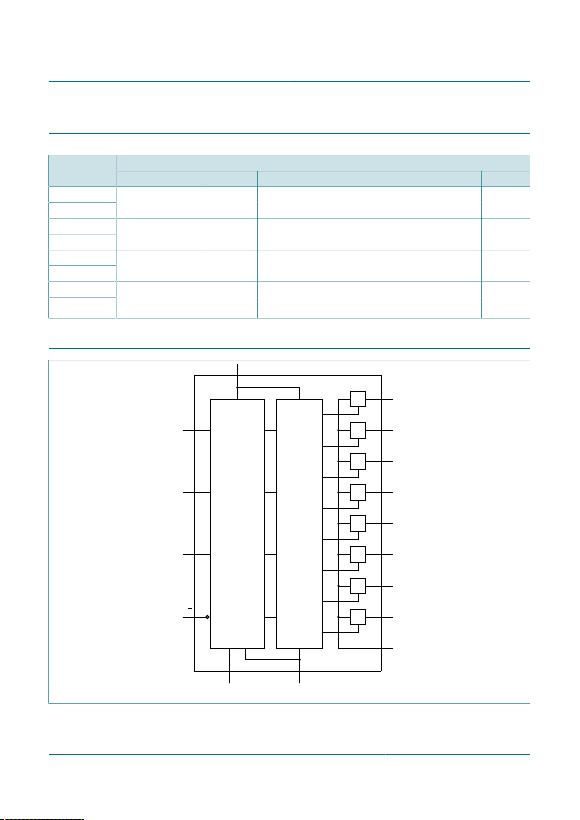Nexperia 74HC4051; 74HCT4051
8-channel analog multiplexer/demultiplexer
Product data sheet Rev. 9 — 26 September 2017
2 / 32
4 Ordering information
Table 1. Ordering information
PackageType number
Temperature range Name Description Version
74HC4051D
74HCT4051D
-40 °C to +125 °C SO16 plastic small outline package; 16 leads;
body width 3.9 mm
SOT109-1
74HC4051DB
74HCT4051DB
-40 °C to +125 °C SSOP16 plastic shrink small outline package; 16 leads;
body width 5.3 mm
SOT338-1
74HC4051PW
74HCT4051PW
-40 °C to +125 °C TSSOP16 plastic thin shrink small outline package; 16 leads;
body width 4.4 mm
SOT403-1
74HC4051BQ
74HCT4051BQ
-40 °C to +125 °C DHVQFN16 plastic dual in-line compatible thermal enhanced
body 2.5 x 3.5 x 0.85 mm
SOT763-1
5 Functional diagram
LOGIC
LEVEL
CONVERSION
11
16
VCC
13 Y0
S0
1-OF-8
DECODER
14 Y1
15 Y2
12 Y3
1 Y4
5 Y5
2 Y6
4 Y7
3 Z
10S1
9S2
6
8 7
GND VEE
E
Figure 1.  Functional diagram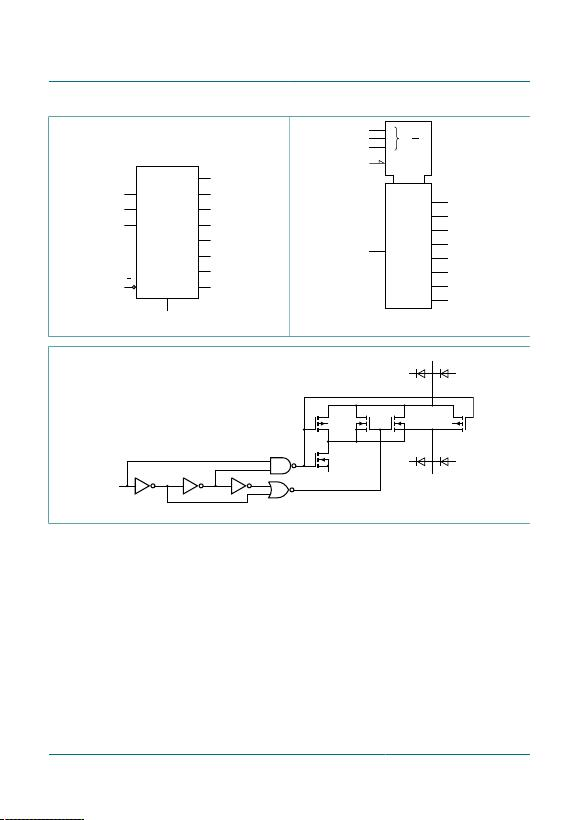Nexperia 74HC4051; 74HCT4051
8-channel analog multiplexer/demultiplexer
Product data sheet Rev. 9 — 26 September 2017
3 / 32
13 Y0
1411 Y1S0
10
S1
9
S2
6
E
15 Y2
12 Y3
1Y4
5Y5
Z
3
2Y6
4Y7
Figure 2.  Logic symbol
2
0
MUX/DMUX 13
G8
14
15
12
3
1
5
2
4
0
1
2
3
4
5
6
7
8 X 0
7
11
10
9
6
Figure 3.  IEC logic symbol
from
logic
VCC
VEE
VEE
VCC
VCC
VEE
Y
Z
VCC
Figure 4.  Schematic diagram (one switch)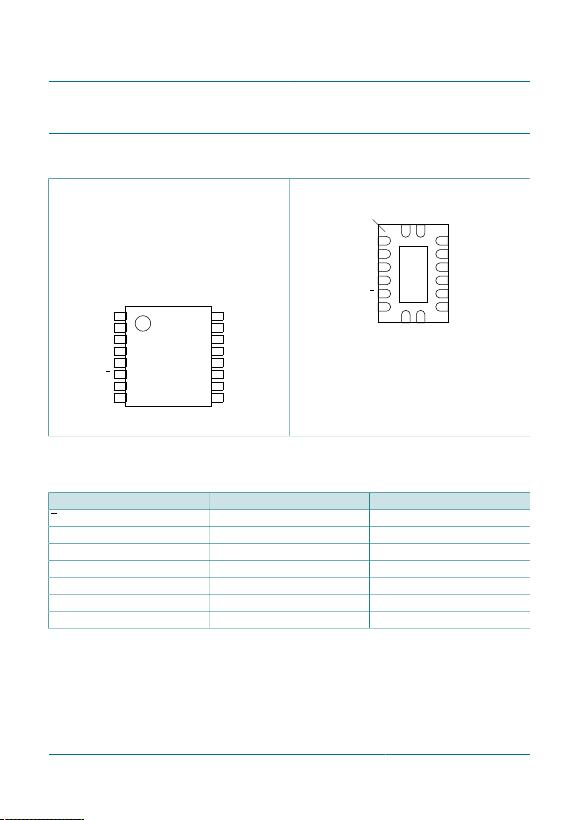Nexperia 74HC4051; 74HCT4051
8-channel analog multiplexer/demultiplexer
Product data sheet Rev. 9 — 26 September 2017
4 / 32
6 Pinning information
6.1 Pinning
Y4 VCC
Y6 Y2
Z Y1
Y7 Y0
Y5 Y3
E S0
VEE S1
GND S2
1
2
3
4
5
6
7
8
10
9
12
11
14
13
16
15
74HC4051
74HCT4051
Figure 5.  Pin configuration SO16, and (T)SSOP16
VEE S1
E S0
Y5 Y3
Y7 Y0
Z Y1
Y6 Y2
GND
S2
Y
4
V
C
C
Transparent top view
7 10
6 11
5 12
4 13
3 14
2 15
8
9
1
16
terminal 1
index area
VCC(1)
74HC4051
74HCT4051
(1) This is not a supply pin. The substrate is attached
to this pad using conductive die attach material. There
is no electrical or mechanical requirement to solder this
pad.However, if it is soldered, the solder land should remain
floating or be connected to VCC.
Figure 6.  Pin configuration DHVQFN16
6.2 Pin description
Table 2. Pin description
Symbol Pin Description
E 6 enable input (active LOW)
VEE 7 supply voltage
GND 8 ground supply voltage
S0, S1, S2 11, 10, 9 select input
Y0, Y1, Y2, Y3, Y4, Y5, Y6, Y7 13, 14, 15, 12, 1, 5, 2, 4 independent input or output
Z 3 common output or input
VCC 16 supply voltageNexperia 74HC4051; 74HCT4051
8-channel analog multiplexer/demultiplexer
Product data sheet Rev. 9 — 26 September 2017
5 / 32
7 Function description
Table 3. Function table 
Input
E S2 S1 S0
Channel ON
L L L L Y0 to Z
L L L H Y1 to Z
L L H L Y2 to Z
L L H H Y3 to Z
L H L L Y4 to Z
L H L H Y5 to Z
L H H L Y6 to Z
L H H H Y7 to Z
H X X X switches off
 H = HIGH voltage level; L = LOW voltage level; X = don’t care.
8 Limiting values
Table 4. Limiting values
In accordance with the Absolute Maximum Rating System (IEC 60134). Voltages are referenced to VSS = 0 V (ground).
Symbol Parameter Conditions Min Max Unit
VCC supply voltage  -0.5 +11.0 V
IIK input clamping current VI < -0.5 V or VI > VCC + 0.5 V - ±20 mA
ISK switch clamping current VSW < -0.5 V or VSW > VCC + 0.5 V - ±20 mA
ISW switch current -0.5 V < VSW < VCC + 0.5 V - ±25 mA
IEE supply current - ±20 mA
ICC supply current - 50 mA
IGND ground current - -50 mA
Tstg storage temperature -65 +150 °C
Ptot total power dissipation SO16, (T)SSOP16, and
DHVQFN16 package
 - 500 mW
P power dissipation per switch - 100 mW
 To avoid drawing VCC current out of terminal Z, when switch current flows into terminals Yn, the voltage drop across the bidirectional switch must not
exceed 0.4 V. If the switch current flows into terminal Z, no VCC current will flow out of terminals Yn, and in this case there is no limit for the voltage drop
across the switch, but the voltages at Yn and Z may not exceed VCC or VEE.
 For SO16 packages: above 70 °C the value of Ptot derates linearly with 8 mW/K.
For SSOP16 and TSSOP16 packages: above 60 °C the value of Ptot derates linearly with 5.5 mW/K.
For DHVQFN16 packages: above 60 °C the value of Ptot derates linearly with 4.5 mW/K.Nexperia 74HC4051; 74HCT4051
8-channel analog multiplexer/demultiplexer
Product data sheet Rev. 9 — 26 September 2017
6 / 32
9 Recommended operating conditions
Table 5. Recommended operating conditions
74HC4051 74HCT4051Symbol Parameter Conditions
Min Typ Max Min Typ Max
Unit
see Figure 7
and Figure 8
VCC - GND 2.0 5.0 10.0 4.5 5.0 5.5 V
VCC supply voltage
VCC - VEE 2.0 5.0 10.0 2.0 5.0 10.0 V
VIinput voltage GND - VCC GND - VCC V
VSW switch voltage VEE - VCC VEE - VCC V
Tamb ambient
temperature
-40 +25 +125 -40 +25 +125 °C
VCC = 2.0 V - - 625 - - - ns/V
VCC = 4.5 V - 1.67 139 - 1.67 139 ns/V
VCC = 6.0 V - - 83 - - - ns/V
Δt/ΔV input transition
rise and fall
rate
VCC = 10.0 V - - 31 - - - ns/V
VCC - VEE (V)
0 1084 62
4
6
2
8
10
0
operating area
VCC - GND
(V)
Figure 7.  Guaranteed operating area as a function of
the supply voltages for 74HC4051
VCC - VEE (V)
0 1084 62
4
6
2
8
10
0
VCC - GND
(V)
operating area
Figure 8.  Guaranteed operating area as a function of
the supply voltages for 74HCT4051Nexperia 74HC4051; 74HCT4051
8-channel analog multiplexer/demultiplexer
Product data sheet Rev. 9 — 26 September 2017
7 / 32
10 Static characteristics
Table 6. RON resistance per switch for 74HC4051 and 74HCT4051
VI = VIH or VIL; for test circuit see Figure 9.
Vis is the input voltage at a Yn or Z terminal, whichever is assigned as an input.
Vos is the output voltage at a Yn or Z terminal, whichever is assigned as an output.
For 74HC4051: VCC - GND or VCC - VEE = 2.0 V, 4.5 V, 6.0 V and 9.0 V.
For 74HCT4051: VCC - GND = 4.5 V and 5.5 V, VCC - VEE = 2.0 V, 4.5 V, 6.0 V and 9.0 V.
Symbol Parameter Conditions Min Typ Max Unit
Tamb = 25 °C
Vis = VCC to VEE
VCC = 2.0 V; VEE = 0 V; ISW = 100 μA  - - - Ω
VCC = 4.5 V; VEE = 0 V; ISW = 1000 μA - 100 180 Ω
VCC = 6.0 V; VEE = 0 V; ISW = 1000 μA - 90 160 Ω
RON(peak) ON resistance (peak)
VCC = 4.5 V; VEE = -4.5 V; ISW = 1000 μA - 70 130 Ω
Vis = VEE
VCC = 2.0 V; VEE = 0 V; ISW = 100 μA  - 150 - Ω
VCC = 4.5 V; VEE = 0 V; ISW = 1000 μA - 80 140 Ω
VCC = 6.0 V; VEE = 0 V; ISW = 1000 μA - 70 120 Ω
VCC = 4.5 V; VEE = -4.5 V; ISW = 1000 μA - 60 105 Ω
Vis = VCC
VCC = 2.0 V; VEE = 0 V; ISW = 100 μA  - 150 - Ω
VCC = 4.5 V; VEE = 0 V; ISW = 1000 μA - 90 160 Ω
VCC = 6.0 V; VEE = 0 V; ISW = 1000 μA - 80 140 Ω
RON(rail) ON resistance (rail)
VCC = 4.5 V; VEE = -4.5 V; ISW = 1000 μA - 65 120 Ω
Vis = VCC to VEE
VCC = 2.0 V; VEE = 0 V  - - - Ω
VCC = 4.5 V; VEE = 0 V - 9 - Ω
VCC = 6.0 V; VEE = 0 V - 8 - Ω
ΔRON ON resistance mismatch
between channels
VCC = 4.5 V; VEE = -4.5 V - 6 - ΩNexperia 74HC4051; 74HCT4051
8-channel analog multiplexer/demultiplexer
Product data sheet Rev. 9 — 26 September 2017
8 / 32
Symbol Parameter Conditions Min Typ Max Unit
Tamb = -40 °C to +85 °C
Vis = VCC to VEE
VCC = 2.0 V; VEE = 0 V; ISW = 100 μA  - - - Ω
VCC = 4.5 V; VEE = 0 V; ISW = 1000 μA - - 225 Ω
VCC = 6.0 V; VEE = 0 V; ISW = 1000 μA - - 200 Ω
RON(peak) ON resistance (peak)
VCC = 4.5 V; VEE = -4.5 V; ISW = 1000 μA - - 165 Ω
Vis = VEE
VCC = 2.0 V; VEE = 0 V; ISW = 100 μA  - - - Ω
VCC = 4.5 V; VEE = 0 V; ISW = 1000 μA - - 175 Ω
VCC = 6.0 V; VEE = 0 V; ISW = 1000 μA - - 150 Ω
VCC = 4.5 V; VEE = -4.5 V; ISW = 1000 μA - - 130 Ω
Vis = VCC
VCC = 2.0 V; VEE = 0 V; ISW = 100 μA  - - - Ω
VCC = 4.5 V; VEE = 0 V; ISW = 1000 μA - - 200 Ω
VCC = 6.0 V; VEE = 0 V; ISW = 1000 μA - - 175 Ω
RON(rail) ON resistance (rail)
VCC = 4.5 V; VEE = -4.5 V; ISW = 1000 μA - - 150 Ω
Tamb = -40 °C to +125 °C
Vis = VCC to VEE
VCC = 2.0 V; VEE = 0 V; ISW = 100 μA  - - - Ω
VCC = 4.5 V; VEE = 0 V; ISW = 1000 μA - - 270 Ω
VCC = 6.0 V; VEE = 0 V; ISW = 1000 μA - - 240 Ω
RON(peak) ON resistance (peak)
VCC = 4.5 V; VEE = -4.5 V; ISW = 1000 μA - - 195 Ω
Vis = VEE
VCC = 2.0 V; VEE = 0 V; ISW = 100 μA  - - - Ω
VCC = 4.5 V; VEE = 0 V; ISW = 1000 μA - - 210 Ω
VCC = 6.0 V; VEE = 0 V; ISW = 1000 μA - - 180 Ω
VCC = 4.5 V; VEE = -4.5 V; ISW = 1000 μA - - 160 Ω
Vis = VCC
VCC = 2.0 V; VEE = 0 V; ISW = 100 μA  - - - Ω
VCC = 4.5 V; VEE = 0 V; ISW = 1000 μA - - 240 Ω
VCC = 6.0 V; VEE = 0 V; ISW = 1000 μA - - 210 Ω
RON(rail) ON resistance (rail)
VCC = 4.5 V; VEE = -4.5 V; ISW = 1000 μA - - 180 Ω
 When supply voltages (VCC - VEE) near 2.0 V the analog switch ON resistance becomes extremely non-linear. When using a supply of 2 V, it is
recommended to use these devices only for transmitting digital signals.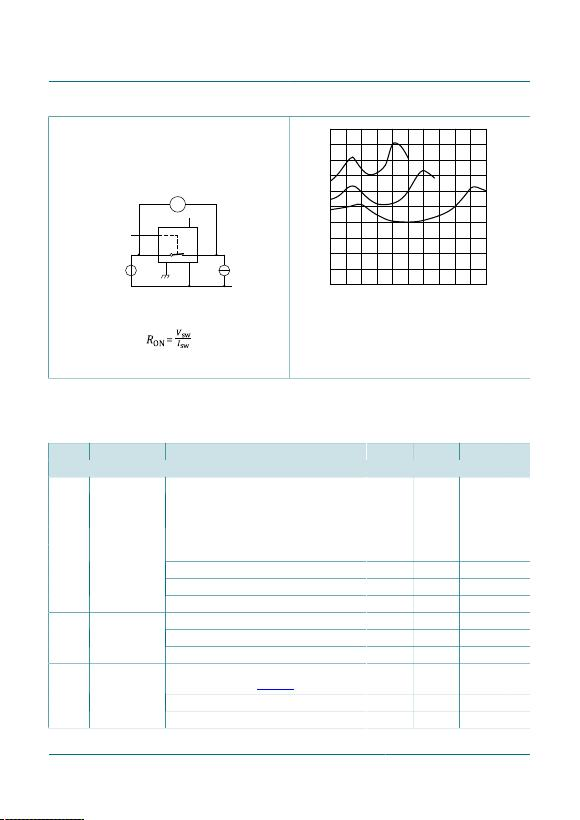Nexperia 74HC4051; 74HCT4051
8-channel analog multiplexer/demultiplexer
Product data sheet Rev. 9 — 26 September 2017
9 / 32
V
001aan389
Yn
Snfrom select
input
Z
GND VEE
VCC
Vis Isw
Vsw
Vis = 0 V to (VCC - VEE).
Figure 9.  Test circuit for measuring RON
Vis (V)
0 9.07.23.6 5.41.8
mnb047
50
70
30
90
110
10
RON
(Ω)
(1)
(2)
(3)
Vis = 0 V to (VCC - VEE).
(1) VCC = 4.5 V
(2) VCC = 6 V
(3) VCC = 9 V
Figure 10.  Typical RON as a function of input voltage Vis
Table 7. Static characteristics for 74HC4051
Voltages are referenced to GND (ground = 0 V).
Vis is the input voltage at pins Yn or Z, whichever is assigned as an input.
Vos is the output voltage at pins Z or Yn, whichever is assigned as an output.
Symbol Parameter Conditions Min Typ Max Unit
Tamb = 25 °C
VCC = 2.0 V 1.5 1.2 - V
VCC = 4.5 V 3.15 2.4 - V
VCC = 6.0 V 4.2 3.2 - V
VIH HIGH-level input
voltage
VCC = 9.0 V 6.3 4.7 - V
VCC = 2.0 V - 0.8 0.5 V
VCC = 4.5 V - 2.1 1.35 V
VCC = 6.0 V - 2.8 1.8 V
VIL LOW-level input
voltage
VCC = 9.0 V - 4.3 2.7 V
VEE = 0 V; VI = VCC or GND
VCC = 6.0 V - - ±0.1 μA
IIinput leakage
current
VCC = 10.0 V - - ±0.2 μA
VCC = 10.0 V; VEE = 0 V; VI = VIH or VIL;
|VSW| = VCC - VEE; see Figure 11
per channel - - ±0.1 μA
IS(OFF) OFF-state
leakage current
all channels - - ±0.4 μANexperia 74HC4051; 74HCT4051
8-channel analog multiplexer/demultiplexer
Product data sheet Rev. 9 — 26 September 2017
10 / 32
Symbol Parameter Conditions Min Typ Max Unit
IS(ON) ON-state leakage
current
VI = VIH or VIL; |VSW| = VCC - VEE;
VCC = 10.0 V; VEE = 0 V; see Figure 12
- - ±0.4 μA
VEE = 0 V; VI = VCC or GND; Vis = VEE or VCC;
Vos = VCC or VEE
VCC = 6.0 V - - 8.0 μA
ICC supply current
VCC = 10.0 V - - 16.0 μA
CIinput capacitance - 3.5 - pF
independent pins Yn - 5 - pFCsw switch
capacitance common pins Z - 25 - pF
Tamb = -40 °C to +85 °C
VCC = 2.0 V 1.5 - - V
VCC = 4.5 V 3.15 - - V
VCC = 6.0 V 4.2 - - V
VIH HIGH-level input
voltage
VCC = 9.0 V 6.3 - - V
VCC = 2.0 V - - 0.5 V
VCC = 4.5 V - - 1.35 V
VCC = 6.0 V - - 1.8 V
VIL LOW-level input
voltage
VCC = 9.0 V - - 2.7 V
VEE = 0 V; VI = VCC or GND
VCC = 6.0 V - - ±1.0 μA
IIinput leakage
current
VCC = 10.0 V - - ±2.0 μA
VCC = 10.0 V; VEE = 0 V; VI = VIH or VIL;
|VSW| = VCC - VEE; see Figure 11
per channel - - ±1.0 μA
IS(OFF) OFF-state
leakage current
all channels - - ±4.0 μA
IS(ON) ON-state leakage
current
VI = VIH or VIL; |VSW| = VCC - VEE;
VCC = 10.0 V; VEE = 0 V; see Figure 12
- - ±4.0 μA
VEE = 0 V; VI = VCC or GND; Vis = VEE or VCC;
Vos = VCC or VEE
VCC = 6.0 V - - 80.0 μA
ICC supply current
VCC = 10.0 V - - 160.0 μANexperia 74HC4051; 74HCT4051
8-channel analog multiplexer/demultiplexer
Product data sheet Rev. 9 — 26 September 2017
11 / 32
Symbol Parameter Conditions Min Typ Max Unit
Tamb = -40 °C to +125 °C
VCC = 2.0 V 1.5 - - V
VCC = 4.5 V 3.15 - - V
VCC = 6.0 V 4.2 - - V
VIH HIGH-level input
voltage
VCC = 9.0 V 6.3 - - V
VCC = 2.0 V - - 0.5 V
VCC = 4.5 V - - 1.35 V
VCC = 6.0 V - - 1.8 V
VIL LOW-level input
voltage
VCC = 9.0 V - - 2.7 V
VEE = 0 V; VI = VCC or GND
VCC = 6.0 V - - ±1.0 μA
IIinput leakage
current
VCC = 10.0 V - - ±2.0 μA
VCC = 10.0 V; VEE = 0 V; VI = VIH or VIL;
|VSW| = VCC - VEE; see Figure 11
per channel - - ±1.0 μA
IS(OFF) OFF-state
leakage current
all channels - - ±4.0 μA
IS(ON) ON-state leakage
current
VI = VIH or VIL; |VSW| = VCC - VEE;
VCC = 10.0 V; VEE = 0 V; see Figure 12
- - ±4.0 μA
VEE = 0 V; VI = VCC or GND; Vis = VEE or VCC;
Vos = VCC or VEE
VCC = 6.0 V - - 160.0 μA
ICC supply current
VCC = 10.0 V - - 320.0 μANexperia 74HC4051; 74HCT4051
8-channel analog multiplexer/demultiplexer
Product data sheet Rev. 9 — 26 September 2017
12 / 32
Table 8. Static characteristics for 74HCT4051
Voltages are referenced to GND (ground = 0 V).
Vis is the input voltage at pins Yn or Z, whichever is assigned as an input.
Vos is the output voltage at pins Z or Yn, whichever is assigned as an output.
Symbol Parameter Conditions Min Typ Max Unit
Tamb = 25 °C
VIH HIGH-level input
voltage
VCC = 4.5 V to 5.5 V 2.0 1.6 - V
VIL LOW-level input
voltage
VCC = 4.5 V to 5.5 V - 1.2 0.8 V
IIinput leakage
current
VI = VCC or GND; VCC = 5.5 V; VEE = 0 V - - ±0.1 μA
VCC = 10.0 V; VEE = 0 V; VI = VIH or VIL;
|VSW| = VCC - VEE; see Figure 11
per channel - - ±0.1 μA
IS(OFF) OFF-state
leakage current
all channels - - ±0.4 μA
IS(ON) ON-state
leakage current
VCC = 10.0 V; VEE = 0 V; VI = VIH or VIL;
|VSW| = VCC - VEE; see Figure 12
- - ±0.4 μA
VI = VCC or GND; Vis = VEE or VCC;
Vos = VCC or VEE
VCC = 5.5 V; VEE = 0 V - - 8.0 μA
ICC supply current
VCC = 5.0 V; VEE = -5.0 V - - 16.0 μA
current
per input; VI = VCC - 2.1 V; other inputs at VCC
or GND; VCC = 4.5 V to 5.5 V; VEE = 0 V
- 50 180 μA
CIinput
capacitance
- 3.5 - pF
independent pins Yn - 5 - pFCsw switch
capacitance common pins Z - 25 - pFNexperia 74HC4051; 74HCT4051
8-channel analog multiplexer/demultiplexer
Product data sheet Rev. 9 — 26 September 2017
13 / 32
Symbol Parameter Conditions Min Typ Max Unit
Tamb = -40 °C to +85 °C
VIH HIGH-level input
voltage
VCC = 4.5 V to 5.5 V 2.0 - - V
VIL LOW-level input
voltage
VCC = 4.5 V to 5.5 V - - 0.8 V
IIinput leakage
current
VI = VCC or GND; VCC = 5.5 V; VEE = 0 V - - ±1.0 μA
VCC = 10.0 V; VEE = 0 V; VI = VIH or VIL;
|VSW| = VCC - VEE; see Figure 11
per channel - - ±1.0 μA
IS(OFF) OFF-state
leakage current
all channels - - ±4.0 μA
IS(ON) ON-state
leakage current
VCC = 10.0 V; VEE = 0 V; VI = VIH or VIL;
|VSW| = VCC - VEE; see Figure 12
- - ±4.0 μA
VI = VCC or GND; Vis = VEE or VCC;
Vos = VCC or VEE
VCC = 5.5 V; VEE = 0 V - - 80.0 μA
ICC supply current
VCC = 5.0 V; VEE = -5.0 V - - 160.0 μA
current
per input; VI = VCC - 2.1 V; other inputs at VCC
or GND; VCC = 4.5 V to 5.5 V; VEE = 0 V
- - 225 μA
Tamb = -40 °C to +125 °C
VIH HIGH-level input
voltage
VCC = 4.5 V to 5.5 V 2.0 - - V
VIL LOW-level input
voltage
VCC = 4.5 V to 5.5 V - - 0.8 V
IIinput leakage
current
VI = VCC or GND; VCC = 5.5 V; VEE = 0 V - - ±1.0 μA
VCC = 10.0 V; VEE = 0 V; VI = VIH or VIL;
|VSW| = VCC - VEE; see Figure 11
per channel - - ±1.0 μA
IS(OFF) OFF-state
leakage current
all channels - - ±4.0 μA
IS(ON) ON-state
leakage current
VCC = 10.0 V; VEE = 0 V; VI = VIH or VIL;
|VSW| = VCC - VEE; see Figure 12
- - ±4.0 μA
VI = VCC or GND; Vis = VEE or VCC;
Vos = VCC or VEE
VCC = 5.5 V; VEE = 0 V - - 160.0 μA
ICC supply current
VCC = 5.0 V; VEE = -5.0 V - - 320.0 μA
current
per input; VI = VCC - 2.1 V; other inputs at VCC
or GND; VCC = 4.5 V to 5.5 V; VEE = 0 V
- - 245 μA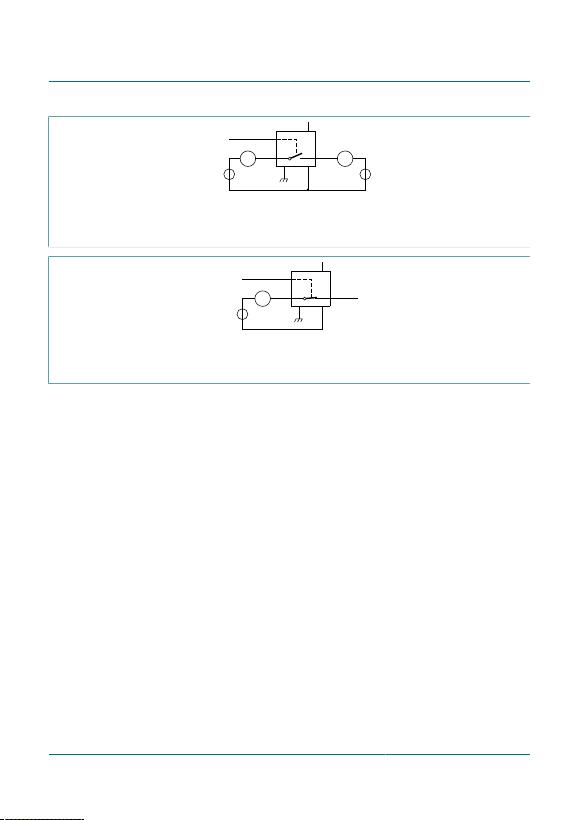Nexperia 74HC4051; 74HCT4051
8-channel analog multiplexer/demultiplexer
Product data sheet Rev. 9 — 26 September 2017
14 / 32
Vis = VCC and Vos = VEE.
Vis = VEE and Vos = VCC.
Figure 11.  Test circuit for measuring OFF-state current
Isw
A
001aah828
nYn
Sn
HIGH
from select
input
nZ
GND VEE
VCC
Vis
Vos
Vis = VCC and Vos = open-circuit.
Vis = VEE and Vos = open-circuit.
Figure 12.  Test circuit for measuring ON-state currentNexperia 74HC4051; 74HCT4051
8-channel analog multiplexer/demultiplexer
Product data sheet Rev. 9 — 26 September 2017
15 / 32
11 Dynamic characteristics
Table 9. Dynamic characteristics for 74HC4051
GND = 0 V; tr = tf = 6 ns; CL = 50 pF; for test circuit see Figure 15.
Vis is the input voltage at a Yn or Z terminal, whichever is assigned as an input.
Vos is the output voltage at a Yn or Z terminal, whichever is assigned as an output.
Symbol Parameter Conditions Min Typ Max Unit
Tamb = 25 °C
Vis to Vos; RL = ∞ Ω; see Figure 13 
VCC = 2.0 V; VEE = 0 V - 14 60 ns
VCC = 4.5 V; VEE = 0 V - 5 12 ns
VCC = 6.0 V; VEE = 0 V - 4 10 ns
tpd propagation delay
VCC = 4.5 V; VEE = -4.5 V - 4 8 ns
E to Vos; RL = ∞ Ω; see Figure 14 
VCC = 2.0 V; VEE = 0 V - 72 345 ns
VCC = 4.5 V; VEE = 0 V - 29 69 ns
VCC = 5.0 V; VEE = 0 V; CL = 15 pF - 22 - ns
VCC = 6.0 V; VEE = 0 V - 21 59 ns
VCC = 4.5 V; VEE = -4.5 V - 18 51 ns
Sn to Vos; RL = ∞ Ω; see Figure 14 
VCC = 2.0 V; VEE = 0 V - 66 345 ns
VCC = 4.5 V; VEE = 0 V - 28 69 ns
VCC = 5.0 V; VEE = 0 V; CL = 15 pF - 20 - ns
VCC = 6.0 V; VEE = 0 V - 19 59 ns
ton turn-on time
VCC = 4.5 V; VEE = -4.5 V - 16 51 ns
E to Vos; RL = 1 kΩ; see Figure 14 
VCC = 2.0 V; VEE = 0 V - 58 290 ns
VCC = 4.5 V; VEE = 0 V - 31 58 ns
VCC = 5.0 V; VEE = 0 V; CL = 15 pF - 18 - ns
VCC = 6.0 V; VEE = 0 V - 17 49 ns
VCC = 4.5 V; VEE = -4.5 V - 18 42 ns
Sn to Vos; RL = 1 kΩ; see Figure 14 
VCC = 2.0 V; VEE = 0 V - 61 290 ns
VCC = 4.5 V; VEE = 0 V - 25 58 ns
VCC = 5.0 V; VEE = 0 V; CL = 15 pF - 19 - ns
VCC = 6.0 V; VEE = 0 V - 18 49 ns
toff turn-off time
VCC = 4.5 V; VEE = -4.5 V - 18 42 nsNexperia 74HC4051; 74HCT4051
8-channel analog multiplexer/demultiplexer
Product data sheet Rev. 9 — 26 September 2017
16 / 32
Symbol Parameter Conditions Min Typ Max Unit
CPD power dissipation
capacitance
per switch; VI = GND to VCC
 - 25 - pF
Tamb = -40 °C to +85 °C
Vis to Vos; RL = ∞ Ω; see Figure 13 
VCC = 2.0 V; VEE = 0 V - - 75 ns
VCC = 4.5 V; VEE = 0 V - - 15 ns
VCC = 6.0 V; VEE = 0 V - - 13 ns
tpd propagation delay
VCC = 4.5 V; VEE = -4.5 V - - 10 ns
E to Vos; RL = ∞ Ω; see Figure 14 
VCC = 2.0 V; VEE = 0 V - - 430 ns
VCC = 4.5 V; VEE = 0 V - - 86 ns
VCC = 6.0 V; VEE = 0 V - - 73 ns
VCC = 4.5 V; VEE = -4.5 V - - 64 ns
Sn to Vos; RL = ∞ Ω; see Figure 14 
VCC = 2.0 V; VEE = 0 V - - 430 ns
VCC = 4.5 V; VEE = 0 V - - 86 ns
VCC = 6.0 V; VEE = 0 V - - 73 ns
ton turn-on time
VCC = 4.5 V; VEE = -4.5 V - - 64 ns
E to Vos; RL = 1 kΩ; see Figure 14 
VCC = 2.0 V; VEE = 0 V - - 365 ns
VCC = 4.5 V; VEE = 0 V - - 73 ns
VCC = 6.0 V; VEE = 0 V - - 62 ns
VCC = 4.5 V; VEE = -4.5 V - - 53 ns
Sn to Vos; RL = 1 kΩ; see Figure 14 
VCC = 2.0 V; VEE = 0 V - - 365 ns
VCC = 4.5 V; VEE = 0 V - - 73 ns
VCC = 6.0 V; VEE = 0 V - - 62 ns
toff turn-off time
VCC = 4.5 V; VEE = -4.5 V - - 53 nsNexperia 74HC4051; 74HCT4051
8-channel analog multiplexer/demultiplexer
Product data sheet Rev. 9 — 26 September 2017
17 / 32
Symbol Parameter Conditions Min Typ Max Unit
Tamb = -40 °C to +125 °C
Vis to Vos; RL = ∞ Ω; see Figure 13 
VCC = 2.0 V; VEE = 0 V - - 90 ns
VCC = 4.5 V; VEE = 0 V - - 18 ns
VCC = 6.0 V; VEE = 0 V - - 15 ns
tpd propagation delay
VCC = 4.5 V; VEE = -4.5 V - - 12 ns
E to Vos; RL = ∞ Ω; see Figure 14 
VCC = 2.0 V; VEE = 0 V - - 520 ns
VCC = 4.5 V; VEE = 0 V - - 104 ns
VCC = 6.0 V; VEE = 0 V - - 88 ns
VCC = 4.5 V; VEE = -4.5 V - - 77 ns
Sn to Vos; RL = ∞ Ω; see Figure 14 
VCC = 2.0 V; VEE = 0 V - - 520 ns
VCC = 4.5 V; VEE = 0 V - - 104 ns
VCC = 6.0 V; VEE = 0 V - - 88 ns
ton turn-on time
VCC = 4.5 V; VEE = -4.5 V - - 77 ns
E to Vos; RL = 1 kΩ; see Figure 14 
VCC = 2.0 V; VEE = 0 V - - 435 ns
VCC = 4.5 V; VEE = 0 V - - 87 ns
VCC = 6.0 V; VEE = 0 V - - 74 ns
VCC = 4.5 V; VEE = -4.5 V - - 72 ns
Sn to Vos; RL = 1 kΩ; see Figure 14 
VCC = 2.0 V; VEE = 0 V - - 435 ns
VCC = 4.5 V; VEE = 0 V - - 87 ns
VCC = 6.0 V; VEE = 0 V - - 74 ns
toff turn-off time
VCC = 4.5 V; VEE = -4.5 V - - 72 ns
 tpd is the same as tPHL and tPLH.
 ton is the same as tPZH and tPZL.
 toff is the same as tPHZ and tPLZ.
 CPD is used to determine the dynamic power dissipation (PD in μW).
PD = CPD x VCC
2 x fi x N + Σ{(CL + Csw) x VCC
2 x fo} where:
fi = input frequency in MHz;
fo = output frequency in MHz;
N = number of inputs switching;
Σ{(CL + Csw) x VCC
2 x fo} = sum of outputs;
CL = output load capacitance in pF;
Csw = switch capacitance in pF;
VCC = supply voltage in V.Nexperia 74HC4051; 74HCT4051
8-channel analog multiplexer/demultiplexer
Product data sheet Rev. 9 — 26 September 2017
18 / 32
Table 10. Dynamic characteristics for 74HCT4051
GND = 0 V; tr = tf = 6 ns; CL = 50 pF; for test circuit see Figure 15.
Vis is the input voltage at a Yn or Z terminal, whichever is assigned as an input.
Vos is the output voltage at a Yn or Z terminal, whichever is assigned as an output.
Symbol Parameter Conditions Min Typ Max Unit
Tamb = 25 °C
Vis to Vos; RL = ∞ Ω; see Figure 13 
VCC = 4.5 V; VEE = 0 V - 5 12 ns
tpd propagation delay
VCC = 4.5 V; VEE = -4.5 V - 4 8 ns
E to Vos; RL = 1 kΩ; see Figure 14 
VCC = 4.5 V; VEE = 0 V - 26 55 ns
VCC = 5.0 V; VEE = 0 V; CL = 15 pF - 22 - ns
VCC = 4.5 V; VEE = -4.5 V - 16 39 ns
Sn to Vos; RL = 1 kΩ; see Figure 14 
VCC = 4.5 V; VEE = 0 V - 28 55 ns
VCC = 5.0 V; VEE = 0 V; CL = 15 pF - 24 - ns
ton turn-on time
VCC = 4.5 V; VEE = -4.5 V - 16 39 ns
E to Vos; RL = 1 kΩ; see Figure 14 
VCC = 4.5 V; VEE = 0 V - 19 45 ns
VCC = 5.0 V; VEE = 0 V; CL = 15 pF - 16 - ns
VCC = 4.5 V; VEE = -4.5 V - 16 32 ns
Sn to Vos; RL = 1 kΩ; see Figure 14 
VCC = 4.5 V; VEE = 0 V - 23 45 ns
VCC = 5.0 V; VEE = 0 V; CL = 15 pF - 20 - ns
toff turn-off time
VCC = 4.5 V; VEE = -4.5 V - 16 32 ns
CPD power dissipation
capacitance
per switch; VI = GND to VCC - 1.5 V  - 25 - pF
Tamb = -40 °C to +85 °C
Vis to Vos; RL = ∞ Ω; see Figure 13 
VCC = 4.5 V; VEE = 0 V - - 15 ns
tpd propagation delay
VCC = 4.5 V; VEE = -4.5 V - - 10 ns
E to Vos; RL = 1 kΩ; see Figure 14 
VCC = 4.5 V; VEE = 0 V - - 69 ns
VCC = 4.5 V; VEE = -4.5 V - - 49 ns
Sn to Vos; RL = 1 kΩ; see Figure 14 
VCC = 4.5 V; VEE = 0 V - - 69 ns
ton turn-on time
VCC = 4.5 V; VEE = -4.5 V - - 49 nsNexperia 74HC4051; 74HCT4051
8-channel analog multiplexer/demultiplexer
Product data sheet Rev. 9 — 26 September 2017
19 / 32
Symbol Parameter Conditions Min Typ Max Unit
E to Vos; RL = 1 kΩ; see Figure 14 
VCC = 4.5 V; VEE = 0 V - - 56 ns
VCC = 4.5 V; VEE = -4.5 V - - 40 ns
Sn to Vos; RL = 1 kΩ; see Figure 14 
VCC = 4.5 V; VEE = 0 V - - 56 ns
toff turn-off time
VCC = 4.5 V; VEE = -4.5 V - - 40 ns
Tamb = -40 °C to +125 °C
Vis to Vos; RL = ∞ Ω; see Figure 13 
VCC = 4.5 V; VEE = 0 V - - 18 ns
tpd propagation delay
VCC = 4.5 V; VEE = -4.5 V - - 12 ns
E to Vos; RL = 1 kΩ; see Figure 14 
VCC = 4.5 V; VEE = 0 V - - 83 ns
VCC = 4.5 V; VEE = -4.5 V - - 59 ns
Sn to Vos; RL = 1 kΩ; see Figure 14 
VCC = 4.5 V; VEE = 0 V - - 83 ns
ton turn-on time
VCC = 4.5 V; VEE = -4.5 V - - 59 ns
E to Vos; RL = 1 kΩ; see Figure 14 
VCC = 4.5 V; VEE = 0 V - - 68 ns
VCC = 4.5 V; VEE = -4.5 V - - 48 ns
Sn to Vos; RL = 1 kΩ; see Figure 14 
VCC = 4.5 V; VEE = 0 V - - 68 ns
toff turn-off time
VCC = 4.5 V; VEE = -4.5 V - - 48 ns
 tpd is the same as tPHL and tPLH.
 ton is the same as tPZH and tPZL.
 toff is the same as tPHZ and tPLZ.
 CPD is used to determine the dynamic power dissipation (PD in μW).
PD = CPD x VCC
2 x fi x N + Σ{(CL + Csw) x VCC
2 x fo} where:
fi = input frequency in MHz;
fo = output frequency in MHz;
N = number of inputs switching;
Σ{(CL + Csw) x VCC
2 x fo} = sum of outputs;
CL = output load capacitance in pF;
Csw = switch capacitance in pF;
VCC = supply voltage in V.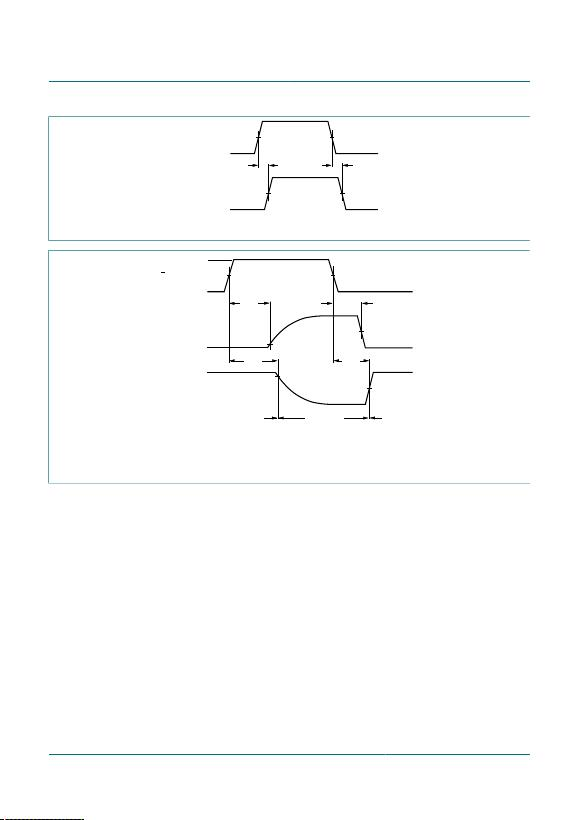Nexperia 74HC4051; 74HCT4051
8-channel analog multiplexer/demultiplexer
Product data sheet Rev. 9 — 26 September 2017
20 / 32
tPLH tPHL
50 %
50 %
Vis input
Vos output
Figure 13.  Input (Vis) to output (Vos) propagation delays
tPLZ
tPHZ
switch OFF switch ON
switch ON
Vos output
Vos output
E, Sn inputs VM
VI
0 V
90 %
10 %
tPZL
tPZH
50 %
50 %
For 74HC4051: VM = 0.5 x VCC.
For 74HCT4051: VM = 1.3 V.
Figure 14.  Turn-on and turn-off times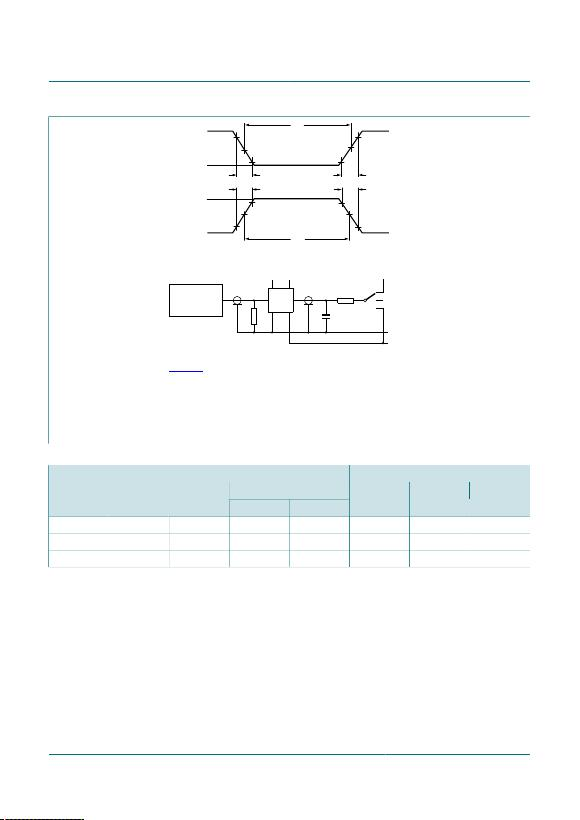Nexperia 74HC4051; 74HCT4051
8-channel analog multiplexer/demultiplexer
Product data sheet Rev. 9 — 26 September 2017
21 / 32
VMVM
tW
tW
10 %
90 %
0 V
VI
VI
negative
pulse
positive
pulse
0 V
VMVM
90 %
10 %
tf
tr
tr
tf
001aae382
VCC VCC
open
GND
VEE
VIVos
DUT
CL
RT
RLS1
PULSE
GENERATOR
Vis
Definitions for test circuit; see Table 11:
RT = termination resistance should be equal to the output impedance Zo of the pulse generator.
CL = load capacitance including jig and probe capacitance.
S1 = Test selection switch.
Figure 15.  Test circuit for measuring switching times
Table 11. Test data
tr, tf
Test
VIVis
at fmax other 
CLRL
S1 position
tPHL, tPLH
 pulse < 2 ns 6 ns 50 pF 1 kΩ open
tPZH, tPHZ
 VCC < 2 ns 6 ns 50 pF 1 kΩ VEE
tPZL, tPLZ
 VEE < 2 ns 6 ns 50 pF 1 kΩ VCC
 tr = tf = 6 ns; when measuring fmax, there is no constraint to tr and tf with 50 % duty factor.
 VI values:
For 74HC4051: VI = VCC
For 74HCT4051: VI = 3 V